TitleIntermediate Algebra
Answer/Discussion to Practice Problems
Tutorial 13: Introduction to FunctionsAnswer/Discussion to 1a {(1, -1), (1, -2), (2, -3), (3, - 4)}   Domain We need to find the set of all input values.  In terms of ordered pairs, that correlates with the first component of each one.  So, what do you get for the domain? If you got {1, 2, 3}, you are correct!     Range We need to find the set of all output values.  In terms of ordered pairs, that correlates with the second component of each one.  So, what do you get for the range? If you got {-1, -2, -3, - 4}, you are absolutely right!   Is this a function or not? We need to ask ourselves, does every first element (or input) correspond with EXACTLY ONE second element (or output)?  In this case the answer is no. 1 corresponds to two y values, -1 and -2. So, this relation would not be an example of a function. (return to problem 1a)Answer/Discussion to 1b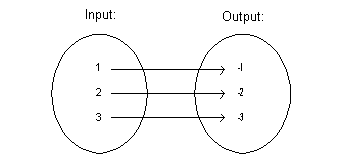Domain We need to find the set of all input values. So, what do you get for the domain? If you got {1, 2, 3}, you are correct!     Range We need to find the set of all output values.  So, what do you get for the range? If you got {-1, -2, -3}, you are absolutely right!     Is this a function or not? We need to ask ourselves, does every first element (or input) correspond with EXACTLY ONE second element (or output)?  In this case the answer is yes. So, this relation would be an example of a function. (return to problem 1b)Answer/Discussion to 2a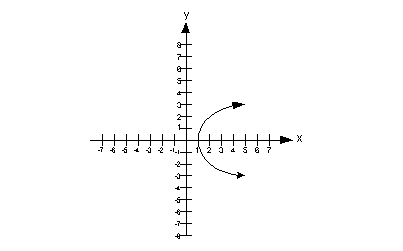Domain We need to find the set of all input values.  In terms of ordered pairs, that correlates with the first component of each one.  In terms of this two dimensional graph, that corresponds with the x values (horizontal axis).  Since that is the case, we need to look to the left and right and see if there are any end points.  In this case, note that the farthest left point is (1, 0) and it keeps going and going to the right.  That means that if we wrote out ordered pairs we would use values that are greater than or equal to 1 for x. This means that the domain is {x | 1 < x}.   Range We need to find the set of all output values.  In terms of ordered pairs, that correlates with the second component of each one. In terms of this two dimensional graph, that corresponds with the y values (vertical axis).  Since that is the case, we need to look up and down and if there are any end points.  In this case, note that the graph keeps going on and on up and down.  This means that the range is {y | y is a real number}     Vertical Line Test This graph would not pass the vertical line test because there is at least one place on it that we could draw a vertical line and intersect it in more than one place.  In fact, there are a lot of vertical lines that we can draw that would intersect it in more than one place, but we only need to show one to say it is not a function.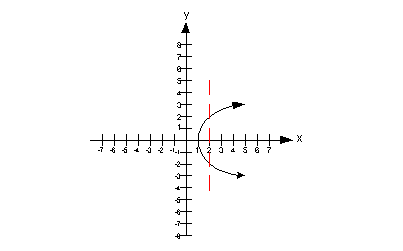Therefore, this is not a graph of a function. (return to problem 2a)Answer/Discussion to 2b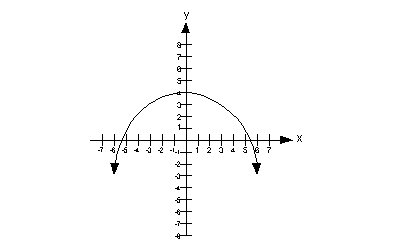Domain We need to find the set of all input values.  In terms of ordered pairs, that correlates with the first component of each one.  In terms of this two dimensional graph, that corresponds with the x values (horizontal axis).  Since that is the case, we need to look to the left and right and see if there are any end points.  In this case, note how the curve has arrows at both ends, that means it would go on and on forever to the right and to the left.  This means that the domain is {x | x is a real number}.     Range We need to find the set of all output values.  In terms of ordered pairs, that correlates with the second component of each one. In terms of this two dimensional graph, that corresponds with the y values (vertical axis).  Since that is the case, we need to look up and down and if there are any end points. In this case, note how the highest point is (0, 4) and it keeps going on and on downward. That means that if we wrote out ordered pairs we would use values that are less than or equal to 4 for y. This means that the range is {y | y < 4}   Vertical Line Test This graph would pass the vertical line test, because there would not be any place on it that we could draw a vertical line and it would intersect it in more than one place. Therefore, this is a graph of a function. (return to problem 2b)Answer/Discussion to 3aSince it is already solved for y squared, to check if y is a function of x, we need to see if there is only one output for every input.At this point, we ask ourselves, would we get one value for y if you plug in any value for x? If you answered no, you are correct.  For example, if our input value x is 9, then our output value y could either be 3 or - 3.  Note that I could have picked an infinite number of examples like this one.  You only need to show one example where the input value is associated with more than one output value to disqualify it from being a function. This means that at least one input value is associated with more than one output value, so that means by definition, y is not a function of x. (return to problem 3a)Answer/Discussion to 3b 2x - 3y = 7   To check if y is a function of x, we need to solve for y first and then check to see if there is only one output for every input.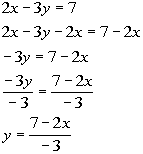*Inverse of add 2x is sub. 2x     *Inverse of mult. by -3 is div. by -3

 At this point we ask ourselves, would we get one value for y if you plug in any value for x? If you answered yes, you are right on.  Note that since it is solved for y, y is our output value and x is our input value.  Since our answer to that question is yes, that means by definition, y is a function of x. (return to problem 3b)Answer/Discussion to 4a  f(x) = 5x + 2     f(-1), f(0), f(.5)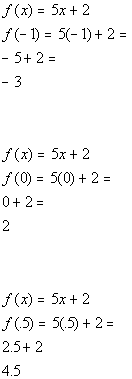*Plug in -1 for x and evaluate           *Plug in 0 for x and evaluate             *Plug in .5 for x and evaluate

 So our answers are f(-1) = -3, f(0) = 2, and f(.5) = 4.5 (return to problem 4a)Answer/Discussion to 4b   g(x) = 2   g(-1), g(0), g(.4)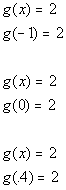*This is a constant function that is always 2     *This is a constant function that is always 2     *This is a constant function that is always 2

 So our answers are g(-1) = 2, g(0) = 2, and g(.4) = 2 (return to problem 4b)

Last revised on July 3, 2011 by Kim Seward.
All contents copyright (C) 2001 - 2011, WTAMU and Kim Seward. All rights reserved.